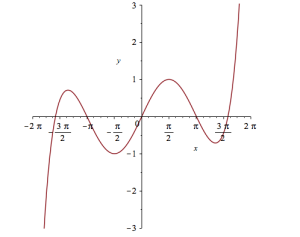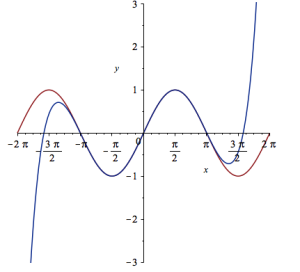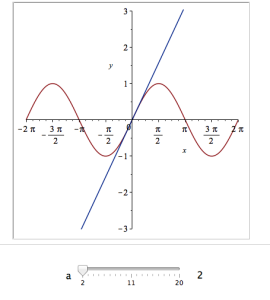## Interactive explorations with Maple: An introduction to the Explore function using the power series for sin(x)

July 13th, 2015 | Categories: Maple, math software, programming, tutorials | Tags:

It is possible to write quick, interactive demonstrations in a variety of languages these days. Functions such as Mathematica’s Manipulate, Sage Math’s interact and IPython’s interact allow programmers to write functional graphical user interfaces with just a few lines of code.

Earlier this week, I hosted a session in the Faculty of Engineering at The University of Sheffield where Maplesoft showed us, among other things, their version of this technology. This blog post is an extension of my notes from this part of the session.

The series command expands a function as a power series around a point. For example, let’s expand sin(x) as a power series around the point x=0.

series(sin(x), x = 0, 10)If we try to plot this, we get an error message

plot(series(sin(x), x = 0, 10), x = -2*Pi .. 2*Pi, y = -3 .. 3)

Warning, unable to evaluate the function to numeric values in the region; see the plotting command's help page to ensure the calling sequence is correct

This is because the output of the series command is a series data structure — something that the plot function cannot handle. We can, however, convert this to a polynomial which is something that the plot function can handle

convert(series(sin(x), x = 0, 10), polynom)Wrapping the above with plot gives:

plot(convert(series(sin(x), x = 0, 10), polynom), x = -2*Pi .. 2*Pi, y = -3 .. 3);Let’s see how close this is to the sin(x) curve by plotting them both together

plot([sin(x), convert(series(sin(x), x = 0, 10), polynom)], x = -2*Pi .. 2*Pi, y = -3 .. 3);It would be nice if we could see how the approximation varies as we vary the number of terms in the expansion. Change the value 10 to a parameter a, pass the whole thing to the Explore function and we get an interactive widget.

Explore(plot([sin(x), convert(series(sin(x), x = 0, a), polynom)], x = -2*Pi .. 2*Pi, y = -3 .. 3), parameters = [a = 2 .. 20]);

Here’s a screenshot of it:It would also be nice to vary the point we expand around. Change the value 0 to b and add an extra parameter to Explore to get two sliders instead of one:

Explore(plot([sin(x), convert(series(sin(x), x = b, a), polynom)], x = -2*Pi .. 2*Pi, y = -3 .. 3), parameters = [a = 2 .. 20, b = -2*Pi .. 2*Pi]);

To see what this looks like, open the companion worksheet in Maple.

We can change the labels on the sliders as follows

Explore(plot([sin(x), convert(series(sin(x), x = b, a), polynom)], x = -2*Pi .. 2*Pi, y = -3 .. 3), parameters = [[a = 2 .. 20, label = Number Of Terms], [b = -2*Pi .. 2*Pi, label = Expansion location]]);

To see what this looks like, open the companion worksheet in Maple.

Finally, let’s set some starting values for each slider

Explore(plot([sin(x), convert(series(sin(x), x = b, a), polynom)], x = -2*Pi .. 2*Pi, y = -3 .. 3), parameters = [[a = 2 .. 20, label = Number Of Terms], [b = -2*Pi .. 2*Pi, label = Expansion location]], initialvalues = [a = 2, b = 1]);

The resulting interactive widget looks like this:Not bad for one line of code!